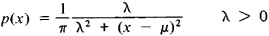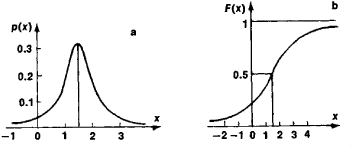# Cauchy Distribution

(redirected from Lorentzian function)

## Cauchy distribution

[kō·shē dis·trə′byü·shən]
(statistics)
A distribution function having the form M /[π M 2+ (x-a)2], where x is the variable and M and a are constants. Also known as Cauchy frequency distribution.

## Cauchy Distribution

a special type of probability distribution of random variables. Introduced by Cauchy, it is marked by the densityThe characteristic function is

f(t) = exp (μit − λ ǀ t ǀ)

The Cauchy distribution is unimodal and symmetric with respect to the point x = μ, which is its mode and median. NoFigure 1. Cauchy distribution: (a) probability density, (b) distribution function

moments of positive order of a Cauchy distribution exist. Figure 1 depicts a Cauchy distribution for μ = 1.5 and λ = 1.

Mentioned in ?
References in periodicals archive ?
The CP histograms, shown in Figure 5, were analyzed by NOVA software using a Lorentzian function. The obtained contact potential average peaks were -80 mV, -260 mV, and -227 mV for samples S1, S2, and S3, respectively, with Full Width at Half Maximum (FWHM) of 0,06-0,09 V for all samples.
where f() is the Lorentzian function and g() is the normal distribution function.
The incoherent scattering function [S.sub.inc](Q, [omega]) in the elastic region is usually separated into an elastic "delta" component, [A.sub.0](Q)[delta]([omega]), and a quasi-elastic component, [A.sub.1](Q)L([omega]), where L([omega]) is a Lorentzian function, centered on [omega] = 0.
Brendel-Bormann reduces the errors generated from the Lorentzian function adopted in Drude by adopting a Gaussian complex error method instead .
In this section, we shall briefly describe INMS method for unconstrained parametric optimization of modified Lorentzian function given by ; (8)
From the grating theory it is known that for the given configuration, the resonance curve is a Lorentzian function [6, 17].
The line profile for stark broadened is well described by a Lorentzian function. Since the instrumental line-broadening exhibit Gaussian shape, then the stark line width [DELTA][[lambda].sub.FWHM] can be extracted from the measured line width [DELTA][[lambda].sub.observed] by subtracting the instrumental line broadening [DELTA][[lambda].sub.instrument]:
The width of the diffraction peaks at half-maximum height was calculated by applying a Lorentzian function to the XRD data, and the crystallite size, D, was estimated by calculating the width of the diffraction peaks according to the Scherrer equation:
Since each resonant mode in a Schumann power spectrum can be approximated by a Lorentzian function [36,37], the magnetic resonant spectrum can be expressed approximately as a superposition of Lorentzian functions obtained by least-squares fitting.
A personal fitting least-square procedure based on a Lorentzian function adjustment is used to process spectral data and decompose the bands into their wavenumber position, absolute intensity, full-width at half maximum and integrated intensity.
Various profile functions can be adopted for crystallite size and microstrain and commonly take the form of a Lorentzian function or a Gaussian function.
Equation (35) is actually a Lorentzian function with peak position and full width at half maximum 2[[pi].sub.[lambda]].

Site: Follow: Share:
Open / Close